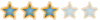# HCF and LCM##HCF and LCM

Code:
`/* Program to print HCF and LCM of 2 numbers*/class HCFandLCM{    public static void lcm(int a, int b)    {     int big=(a>b)?a:b;    //Bigger number    int small=(a>b)?b:a; // smaller number        for(int i=1;i<=big;i++)        {            if(((big*i)%small)==0)            {                int lcm=big*i;                System.out.println("The least common multiple is "+(lcm));                break;            }        }    hcf(big, small);  // Arguments sent to hcf() function    }    public static void hcf(int bigC, int smallC)     {        int temp=1;        while(temp!=0)        {            temp=bigC%smallC;            if(temp==0)            {                System.out.println("Highest Common Factor is "+smallC);            }            else            {                bigC=smallC;                smallC=temp;            }        }    }    public static void main(String[] args)     {    int x=Integer.parseInt(args);    int y=Integer.parseInt(args);    lcm(x, y);    }}`

Variable Table

 Variable Name Data Type Description a,b,bigC,smallC int Formal Parameters big, small int Used to calculate the hcf and lcm x,y int Inputs, Actual Parameters temp int Temporary variable

______________________________________________________
It is the simplest things that are most Complicated!
Been on this forum since 3305 days!

Sh~1457Sujith
LSF ExpertPosts : 126Permissions in this forum:
You cannot reply to topics in this forum